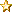###Author Topic: Question about Boolean Algebra  (Read 19 times)

#### TromFan

• Jr. Member
•• Posts: 64
• Karma: +10/-0« on: February 14, 2020, 12:24:17 pm »
I am now reading Insanity Point for about the third or fourth time now.  Each time I read it, the Boolean Algebra makes a little more sense to me.  Between my TROM studies and doing the actual processing, I have developed an intuitive understanding of it and what the phenomena is, and could probably explain it pretty well in plain English, but still, at times it's a headache for me trying to figure out what the actual symbols are intended to mean.

I think if I can understand this ONE THING I believe either it will all make sense or at least I will know what question to answer next.

Here's the passage, followed by my question:

"Now this postulate X(1-X)=1 has some very interesting deductions, very interesting deductions. I'll give them to you. I won't prove these deductions but they can be, I can assure you, every one I'm giving to you can be proven very easily in Boolean algebra.
X(1-X)=1, X is and is not simultaneously
Here we go. We can deduce from X(1-X)=1 that X +(1-X)=0.
X +(1-X)=0, neither X exists nor not X exists.
In other words it's a state of affairs where neither X exists nor not X exists. Get it?
X + (1-X)=1, either X exists or not X exist or both exist
X +(1-X)=0 now that's a state of unreason because reason maintains that X +(1-X)=1 that's what reason maintains.
But unreason, insanity, the IP, says that X + (1-X)=0
X +(1-X)=0, neither X exists nor not X exists.
Now this is a particularly interesting deduction from our point of view because it tells us that while the person is in the IP state the reasonable part of the postulate set is reduced to zero. "

So, what is the difference between X(1-X) and X + (1-X)?  More specifically,  what is X(1-X) supposed to mean?  I took regular algebra in school and I know that when you put two values together like this, it is supposed to mean multiplication,  But I know he does not mean that here.  I looked and I know that with a plus sign it means "and" or "or" but Dennis does not really explain what it means if there is no symbol between the two values.

I have a vague understanding that it means both values simultaneously, but not added, but I am not 100% sure.

I know someone on this forum has to understand this.  I'm no logician.  I have other strong points, but that is not one of them.  Any help explaining is greatly appreciated.